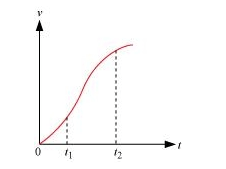# The velocity-time graph of a particle in one-dimensional

Question.
The velocity-time graph of a particle in one-dimensional motion is shown in Fig. 3.29:Which of the following formulae are correct for describing the motion of the particle over the time-interval $t_{2}$ to $t_{1}$ ?

(a) $x\left(t_{2}\right)=x\left(t_{1}\right)+v\left(t_{1}\right)\left(t_{2}-t_{1}\right)+\left(\frac{1}{2}\right) a\left(t_{2}-t_{1}\right)^{2}$

(b) $v\left(t_{2}\right)=v\left(t_{1}\right)+a\left(t_{2}-t_{1}\right)$

(c) $v_{\text {Average }}=\left(x\left(t_{2}\right)-x\left(t_{1}\right)\right) /\left(t_{2}-t_{1}\right)$

(d) $a_{\text {Average }}=\left(v\left(t_{2}\right)-v\left(t_{1}\right)\right) /\left(t_{2}-t_{1}\right)$

(e) $x\left(t_{2}\right)=x\left(t_{1}\right)+v_{\text {Average }}\left(t_{2}-t_{1}\right)+\left(\frac{1}{2}\right) a_{\text {Average }}\left(t_{2}-t_{1}\right)^{2}$

(f) $x\left(t_{2}\right)-x\left(t_{1}\right)=$ area under the $v-t$ curve bounded by the $t$-axis and the dotted line shown.

solution:

The correct formulae describing the motion of the particle are (c), (d) and, (f)

The given graph has a non-uniform slope. Hence, the formulae given in (a), (b), and (e) cannot describe the motion of the particle. Only relations given in (c), (d), and (f) are correct equations of motion.